International
Tables for
Crystallography
Volume F
Crystallography of biological macromolecules
Edited by E. Arnold, D. M. Himmel and M. G. Rossmann

International Tables for Crystallography (2012). Vol. F, ch. 2.2, p. 73

## Section 2.2.11. The most commonly used quality indicators

H. M. Einspahra* and M. S. Weissb

aPO Box 6483, Lawrenceville, NJ 08648–0483, United States, and bHelmholtz-Zentrum Berlin für Materialien und Energie, Macromolecular Crystallography (HZB-MX), Albert-Einstein-Str. 15, D-12489 Berlin, Germany
Correspondence e-mail:  hmeinspahr@yahoo.com

### 2.2.11. The most commonly used quality indicators

| top | pdf |

A summary of the most commonly used quality indicators and their definitions is presented in Table 2.2.11.1for ready reference.

 Table 2.2.11.1| top | pdf | Definitions of the most commonly used quality indicators
IndicatorDetails
Optical resolution,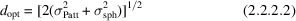σPatt and σsph are the standard uncertainties of Gaussians fitted to the origin peak of the Patterson function of the diffraction data set and the origin peak of the spherical interference function, respectively

Rmerge (merging R factor),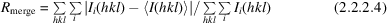<I(hkl)> is the mean of the several individual measurements Ii(hkl) of the intensity of reflection hkl

Rmeas or Rr.i.m (redundancy-independent merging R factor),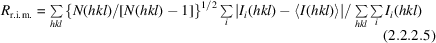<I(hkl)> is the mean of the N(hkl) individual measurements Ii(hkl) of the intensity of reflection hkl

Rp.i.m. (precision-indicating merging R factor),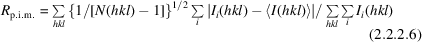<I(hkl)> is the mean of the N(hkl) individual measurements Ii(hkl) of the intensity of reflection hkl

Ranom (anomalous R factor),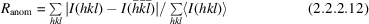<I(hkl)> is the mean intensity of the Friedel mates of the reflection hkl, or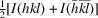RCullis (Cullis R factor for isomorphous-replacement applications),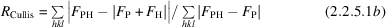FP, FPH and FH are the structure factors for the protein, the heavy-atom derivative and the heavy atoms alone, respectively

PPiso (phasing power for isomorphous-replacement applications),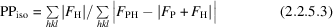or, as in the program SOLVE,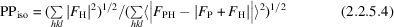FP, FPH and FH are the structure factors for the protein, the heavy-atom derivative and the heavy atoms alone, respectively

R (crystallographic R factor) and Rfree (free R factor),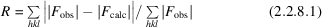Rfree is defined as the crystallographic R factor but for a subset of reflections that have been excluded from refinement

RSRF (real-space R factor),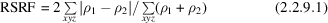ρ1 and ρ2 are the electron-density maps calculated from the structural model and from the experimental data, respectively

R.m.s.d.'s of geometric parameters x,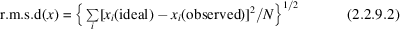xi are the individual values, ideal or observed, of the geometric parameter x and the sum is over all N xi observed. The geometric parameters x may be bond lengths, bond angles, dihedral angles, chiral volumes, deviations from planarity etc.

DPI (diffraction-component precision index),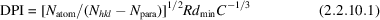Natom is the number of atoms in the structure, Nhkl is the number of reflections, Npara is the number of refined parameters, R is the crystallographic R factor, dmin is the nominal resolution and C is the fractional completeness of the data set